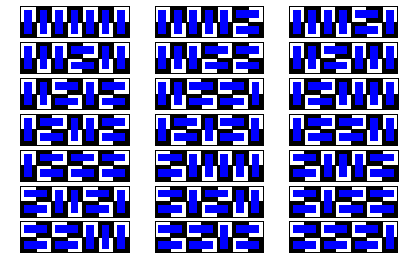# Fibonacci Numbers

The Fibonacci numbers Fn are defined recursively by the relation Fn = Fn−1 + Fn−2, where F1 = F2 = 1. The first few Fibonacci numbers are 1, 1, 2, 3, 5, 8, 13, 21, 34, 55, 89, 144, 233, 377, ...

They describe, among other things, the number of ways to tile a 2 × (n−1) checkerboard with 2 × 1 dominoes.

There’s only one way to tile a 2 × 1 board:Two ways to tile a 2 × 2 board: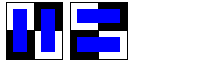Three ways to tile a 2 × 3 board: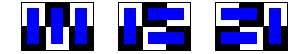Five ways to tile a 2 × 4 board: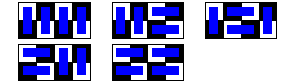Eight ways to tile a 2 × 5 board: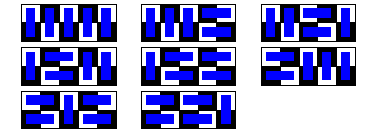Thirteen ways to tile a 2 × 6 board:Twenty-one ways to tile a 2 × 7 board: